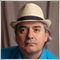# MACD of another indicator85

Hello guys,

i managed to add a MA to an indicator, using the MAOnBuffer,

now i need to create a MACD of the same buffer, so i want to have an MA and a MACD on the same indicator

i tried the iMACD, but i'm getting weird results, i'm not sure if iMACD can be applied to indictor buffers

anyone can give a hint or help with this issue ??

Thanks!Moderator
31179

pipspiper:

Hello guys,

i managed to add a MA to an indicator, using the MAOnBuffer,

What is  MAOnBuffer?85

Keith Watford:

What is  MAOnBuffer?

```#include <MovingAverages.mqh>

switch(*MA MODE*)
{
case MODE_EMA:
ExponentialMAOnBuffer(rates_total,prev_calculated,begin,*MA period*,*indicator buffer*,*MA buffer*);
break;
case MODE_LWMA:
LinearWeightedMAOnBuffer(rates_total,prev_calculated,begin,*MA period*,*indicator buffer*,*MA buffer*);
break;
case MODE_SMA:
SimpleMAOnBuffer(rates_total,prev_calculated,begin,*MA period*,*indicator buffer*,*MA buffer*);
break;
case MODE_SMMA:
SmoothedMAOnBuffer(rates_total,prev_calculated,begin,*MA period*,*indicator buffer*,*MA buffer*);
break;
}```85

Any ideas guys ???6519

pipspiper: Any ideas guys ???

If you study how the MACD is calculated, then you would know. Do some homework and research.

MACD is difference between a fast and a slow EMA, and the signal is an EMA of the difference.85

Fernando Carreiro:

If you study how the MACD is calculated, then you would know. Do some homework and research.

MACD is difference between a fast and a slow EMA, and the signal is an EMA of the difference.

Thanks, ur comment actually solved the problem